# Dehn invariant

(diff) ← Older revision | Latest revision (diff) | Newer revision → (diff)
Jump to: navigation, search

An invariant of polyhedra in three-dimensional space that decides whether two polyhedra of the same volume are "scissors congruent" (see Equal content and equal shape, figures of; Hilbert problems; Polyhedron).

Quite generally, a scissors-congruence invariant assigns to a polytopein space an element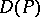in a group such that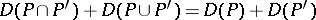,ifis degenerate, and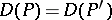if there is a motionof the space such that.

For the Dehn invariant, the group chosen is the tensor product. To a polytopewith edgesone associates the element, where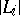is the length of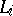and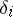is the dihedral angle of the planes meeting at. The Sydler theorem states that two polytopes in three-dimensional space are scissors equivalent if and only if they have equal volume and the same Dehn invariant, thus solving Hilbert's third problem in a very precise manner (cf. also Hilbert problems).

For higher dimensions there is a generalization, called the Hadwiger invariant or Dehn–Hadwiger invariant. This has given results in dimension four, [a4], and for the case when the group consists of translations only, [a2].